Courses
Courses for Kids
Free study material
Free LIVE classes
More

# Word Problems on Multiplication of DecimalsLIVE
Join Vedantu’s FREE Mastercalss

## How to Solve Decimal Word Problems

Before learning about the multiplication of decimal numbers, sit back and think about where you saw decimals earlier. Have you seen it while buying your favourite candy? Probably! Have you seen it on the weighing scale while buying potatoes? Maybe! A decimal is a proper fraction whose denominator is a power of 10. Such fractions are represented in the form of numbers, which can be identified by a (.) full stop in between the digits. Let’s learn about decimal numbers and the process of decimal multiplication.

## Decimal Numbers

As said above, decimal numbers are numbers that have numbers like 10, 100, and 1000 in the denominator. Have a look below at the image.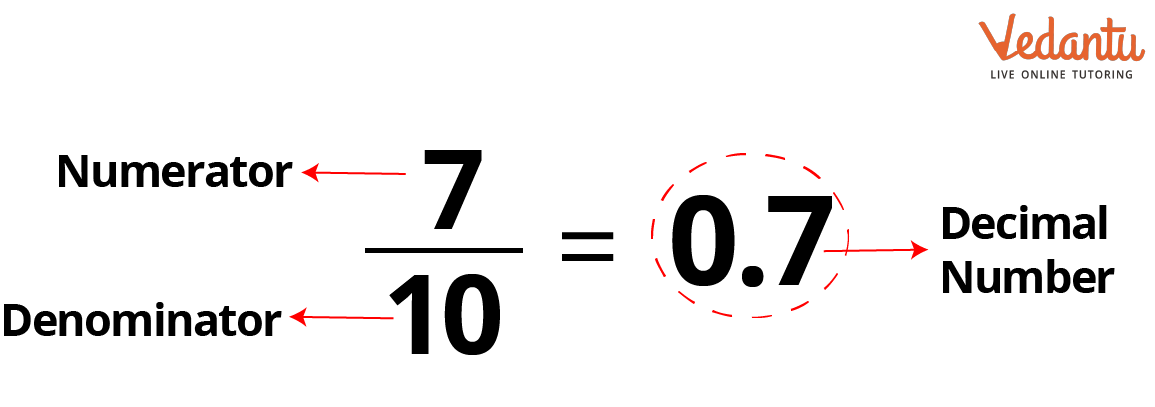Decimal number representation

Let’s learn how to perform the multiplication of such decimal numbers.

## Rules to Solve Problems on Multiplication of Decimal Numbers

Solving decimal addition and subtraction is easy, as you do not have to change the decimal place. However, you have to change the place of the decimal when you perform multiplication or division on decimal numbers or numbers. Let’s understand decimal multiplication word problems with a practical example.

Suppose you went to buy your favourite candy, which costs Rs. 0.50 for one piece. You want to buy four candies. So how will you calculate the amount you have to pay the shopkeeper?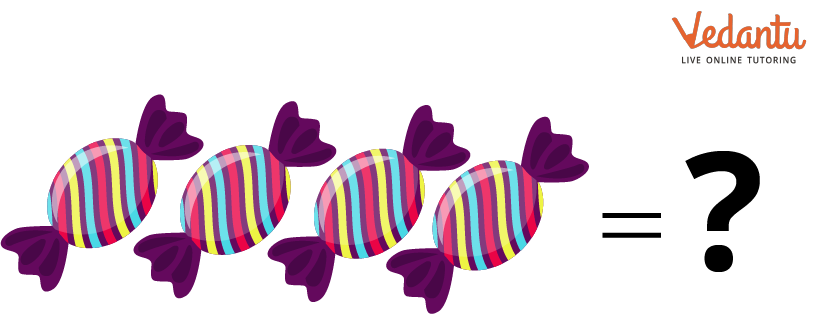Four candies

So, you can calculate the amount by multiplication. You can see that the full stop, or period symbol, between 0 and 5 makes the number decimal. So here, you have to multiply. To perform decimal multiplication, you have to follow certain rules.

Here are the rules you must follow:

1. First, multiply the number by ignoring the decimal place.

2. The next step is to place the decimal point in the product after counting the number of digits before the decimal point in the multiplicand.

Let’s solve the candy problem by using these two rules.

The price of one piece = Rs. 0.50

The price of four pieces will be the price of one piece multiplied by 4, which is =$0.50 \times 4$.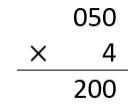First step of decimal multiplication

The next step is to place the decimal point by counting the number of digits before the decimal point in the multiplicand. Here, the multiplicand is 0.50. Hence, we place the decimal point by putting the decimal point after 0 in the product.

Note: Always start from the right side of the digits in the product.

There are two digits in front of the decimal point in the multiplicand. Hence, the result will be Rs. 2.00 as shown below.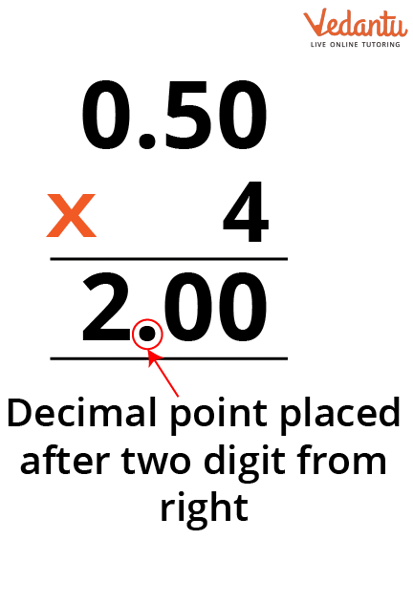Decimal placement after multiplication

So, this is how you can perform multiplication on decimal numbers.

## Solved Examples of Decimal Multiplication

1. Find the product of 12.25 and 2.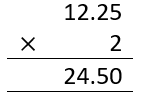Here, the multiplicand contains two digits after the decimal point. So in the solution, the product also has the decimal place after two digits from the right.

1. Determine the product of 14.3 and 4.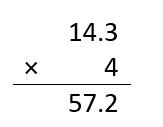Here, the multiplicand contains two digits after the decimal point. So in the solution, the product also has the decimal place after two digits from the right.

1. Find the product of 25.5 and 2.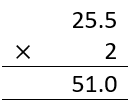Here, the multiplicand contains one digit after the decimal point. So, in the solution, the product also has the decimal place after one digit from the right.

## Decimal Multiplication Worksheet

The questions in the decimal multiplication worksheet given below, along with the answers, will help you practice decimal multiplication much better.

• Find the product of 42.5 and 4

Ans:  170.00

• Find the product of 20.5 and 3

Ans:  61.50

• Find the product of 10.5 and 6

Ans:  63.00

• Find the product of 23.1 and 10

Ans:  231.00

## Conclusion

In this article, you have learnt about decimal numbers. The uses of decimals in daily life and the rules that must be followed while performing decimal multiplication. You have learnt that when performing decimal multiplication, you must take care of the number of digits present in front of the decimal point. Apart from these, you have also learnt to solve decimal multiplication questions.

Last updated date: 01st Oct 2023
Total views: 68.4k
Views today: 0.68k

## FAQs on Word Problems on Multiplication of Decimals

1. Can we multiply a decimal fraction with a decimal fraction?

Yes! We can multiply a decimal fraction by another decimal fraction. However, while writing the result, we have to consider the digits present before the decimal point in both the multiplicand and multiplier. Suppose the multiplicand has two digits in front of the decimal point, and the multiplier has one digit in front of the decimal point. Then the product will have three digits in front of the decimal point.

2. What happens if we place the decimal point by starting from the left?

If you start from the product's left side after multiplication, the answer you will get at the end will be wrong. Therefore, to avoid such mistakes, remember to start from the right while counting the digits to place the decimal points.

3. Why is it important to learn to solve word problems involving decimal multiplication?

It is important to learn decimal multiplication word problems because decimals appear when dealing with money, length, and weight. For example, while measuring your body weight, you must have noticed the numbers sometimes come in decimals. There are many examples of places where decimals are used. Therefore, decimal multiplications are necessary. In fact, all the arithmetic operations on decimal numbers are necessary.